# Commutator relations of field operators

Here is the question:
By using the equality (for boson)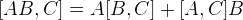---------------------------------------- (1)
Prove that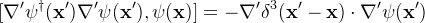Background:

Currently I'm learning things about second quantization in the book "Advanced Quantum Mechanics"(Franz Schwabl).
Given the creation and annihilation operators(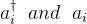), define field operators as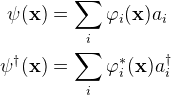The following 3 commutator relations are for Boson.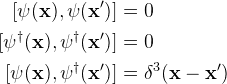-----------------------------------(2)

And here is my attempt (but it doesn't work):

First step, using equality (1) to expand the commutator: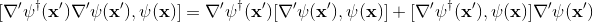-------------(3)
since the nabla operator is an operator, so I think the first term of (3)'s right-hand-side can be expressed as following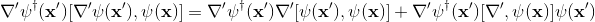also, I expressed the second term of (3)'s right-hand-side by using the same method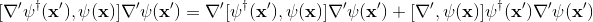So, by inserting those commutators in (2), I found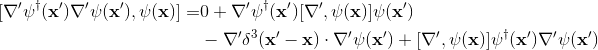Last edited:

strangerep